## 机器学习中的梯度下降法

• 什么是梯度下降法？
• 如何将梯度下降法运用到线性回归模型中？
• 如何利用梯度下降法处理大规模的数据？
• 梯度下降法的一些技巧coefficient=coefficient−(alpha∗delta) coefficient=coefficient−(alpha∗delta) 重复执行上述过程，直到参数值收敛，这样我们就能获得函数的最优解。

• 绘制成本函数随时间变化的曲线：收集并绘制每次迭代过程中所得到的成本函数值。对于梯度下降法来说，每次迭代计算都能降低成本函数值。如果无法降低成本函数值，那么可以尝试减少学习效率值。
• 学习效率：梯度下降算法中的学习效率值通常为0.1，0.001或者0.0001。你可以尝试不同的值然后选出最佳学习效率值。
• 标准化处理：如果成本函数不是偏态形式的话，那么梯度下降法很快就能收敛。隐蔽你可以事先对输入变量进行标准化处理。
• 绘制成本均值趋势图：随机梯度下降法的更新过程通常会带来一些随机噪声，所以我们可以考虑观察10次、100次或1000次更新过程误差均值变化情况来度量算法的收敛趋势。

• 最优化理论是机器学习中非常重要的一部分。
• 梯度下降法是一个简单的最优化算法，你可以将它运用到许多机器学习算法中。
• 批量梯度下降法先计算所有参数的导数值，然后再执行参数更新过程。
• 随机梯度下降法是指从每个训练实例中计算出导数并执行参数更新过程。

## 一文读懂机器学习，大数据/自然语言处理/算法全有了## 1、一个故事说明什么是机器学习

#### 依据数据所做的判断跟机器学习的思想根本上是一致的。刚才的思考过程我只考虑“频次”这种属性。在真实的机器学习中，这可能都不算是一个应用。一般的机器学习模型至少考虑两个量：一个是因变量，也就是我们希望预测的结果，在这个例子里就是小Y迟到与否的判断。另一个是自变量，也就是用来预测小Y是否迟到的量。假设我把时间作为自变量，譬如我发现小Y所有迟到的日子基本都是星期五，而在非星期五情况下他基本不迟到。于是我可以建立一个模型，来模拟小Y迟到与否跟日子是否是星期五的概率。见下图：## 2、机器学习的定义1、房价模型是根据拟合的函数类型决定的。如果是直线，那么拟合出的就是直线方程。如果是其他类型的线，例如抛物线，那么拟合出的就是抛物线方程。机器学习有众多算法，一些强力算法可以拟合出复杂的非线性模型，用来反映一些不是直线所能表达的情况。

2、如果我的数据越多，我的模型就越能够考虑到越多的情况，由此对于新情况的预测效果可能就越好。这是机器学习界“数据为王”思想的一个体现。一般来说(不是绝对)，数据越多，最后机器学习生成的模型预测的效果越好。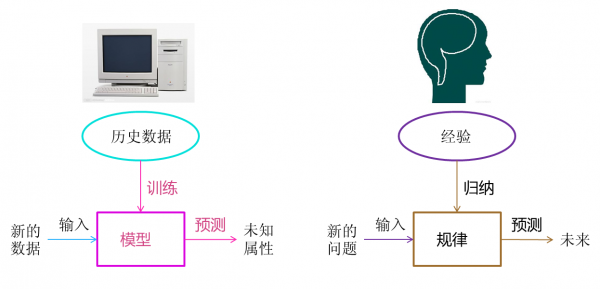#### 3、机器学习的范围## 4、机器学习的方法

#### 1、回归算法#### 2、神经网络#### 3、SVM（支持向量机）## 5、机器学习的应用–大数据1、大数据，小分析：即数据仓库领域的OLAP分析思路，也就是多维分析思想。

2、大数据，大分析：这个代表的就是数据挖掘与机器学习分析法。

3、流式分析：这个主要指的是事件驱动架构。

4、查询分析：经典代表是NoSQL数据库。## 6、机器学习的子类–深度学习

1、多隐层的神经网络具有优异的特征学习能力，学习得到的特征对数据有更本质的刻画，从而有利于可视化或分类；

#### 2、深度神经网络在训练上的难度，可以通过“逐层初始化” 来有效克服。• 2012年6月 ，《纽约时报》披露了Google Brain项目，这个项目是由Andrew Ng和Map-Reduce发明人Jeff Dean共同主导，用16000个CPU Core的并行计算平台训练一种称为“深层神经网络”的机器学习模型，在语音识别和图像识别等领域获得了巨大的成功。Andrew Ng就是文章开始所介绍的机器学习的大牛(图1中右者)。
• 2012年11月， 微软在中国天津的一次活动上公开演示了一个全自动的同声传译系统，讲演者用英文演讲，后台的计算机一气呵成自动完成语音识别、英中机器翻译，以及中文语音合成，效果非常流畅，其中支撑的关键技术是深度学习；
• 2013年1月 ，在百度的年会上，创始人兼CEO李彦宏高调宣布要成立百度研究院，其中第一个重点方向就是深度学习，并为此而成立深度学习研究院(IDL)。
• 2013年4月 ，《麻省理工学院技术评论》杂志将深度学习列为2013年十大突破性技术(Breakthrough Technology)之首。## 7、机器学习的父类–人工智能## 10、后记

Paesey  McParseface 建立于强大的机器学习算法，可以学会分析句子的语言结构，能解释特定句子中每一个词的功能。此类模型中，Paesey  McParseface是世界上最精确的，我们希望他能帮助对自动提取信息、翻译和其它自然语言理解（NLU）中的应用感兴趣的研究者和开放者。

SyntaxNet是怎么工作的？

SyntaxNet是一个框架，即学术圈所指的SyntacticParser，他是许多NLU系统中的关键组件。在这个系统中输入一个句子，他会自动给句子中的每一个单词打上POS（part-of-Speech）标签，用来描述这些词的句法功能，并在依存句法树中呈现。这些句法关系直接涉及句子的潜在含义。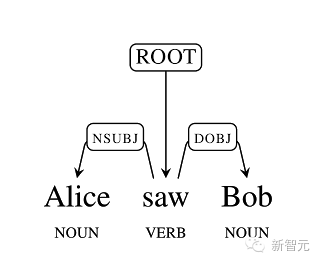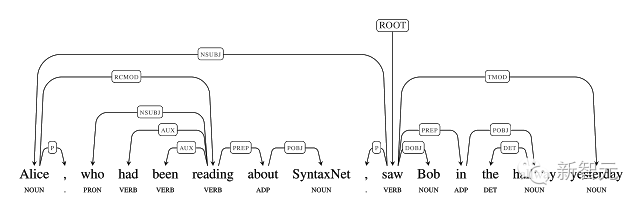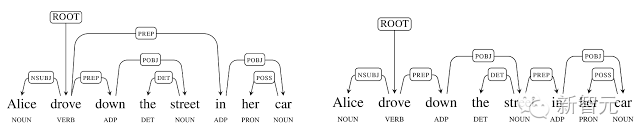SyntaxNet 将神经网络运用于歧义问题。一个输入句子被从左到右地处理。当句子中的每个词被处理时，词与词之间的依存关系也会被逐步地添加进来。由于歧义的存在，在处理过程的每个时间点上都存在多种可能的决策，而神经网络会基于这些决策的合理性向这些彼此竞争的决策分配分数。出于这一原因，在该模型中使用 Beam Search （集束搜索）就变得十分重要。不是直接取每个时间点上的最优决定，而是在每一步都保留多个部分性假设。只有当存在多个得分更高的假设的时候，一个假设才会被抛弃。下图将展示的，是“I booked a ticket to Google”这句话经过从左到右的决策过程而产生的简单句法分析。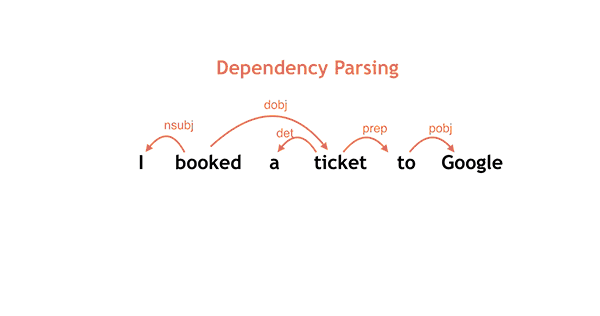Parsey McParseface 的准确度到底有多高？

By:kubiCode

## 有监督学习和无监督学习的区别

• 有监督学习：对具有标记的训练样本进行学习，以尽可能对训练样本集外的数据进行分类预测。（LR,SVM,BP,RF,GBRT）
• 无监督学习：对未标记的样本进行训练学习，比发现这些样本中的结构知识。(KMeans,DL)

## 过拟合

### 产生的原因

1. 因为参数太多，会导致我们的模型复杂度上升，容易过拟合
2. 权值学习迭代次数足够多(Overtraining),拟合了训练数据中的噪声和训练样例中没有代表性的特征.

1. 交叉验证法
2. 减少特征
3. 正则化
4. 权值衰减
5. 验证数据

## 生成模型和判别模型

1. 生成模型：由数据学习联合概率分布P(X,Y)，然后求出条件概率分布P(Y|X)作为预测的模型，即生成模型：P(Y|X)= P(X,Y)/ P(X)。（朴素贝叶斯）
生成模型可以还原联合概率分布p(X,Y)，并且有较快的学习收敛速度，还可以用于隐变量的学习
2. 判别模型：由数据直接学习决策函数Y=f(X)或者条件概率分布P(Y|X)作为预测的模型，即判别模型。（k近邻、决策树）
直接面对预测，往往准确率较高，直接对数据在各种程度上的抽象，所以可以简化模型

## 线性分类器与非线性分类器的区别以及优劣

SVM两种都有(看线性核还是高斯核)

• 线性分类器速度快、编程方便，但是可能拟合效果不会很好
• 非线性分类器编程复杂，但是效果拟合能力强

## L1和L2正则的区别，如何选择L1和L2正则

• L1是在loss function后面加上 模型参数的1范数（也就是|xi|）
• L2是在loss function后面加上 模型参数的2范数（也就是sigma(xi^2)），注意L2范数的定义是sqrt(sigma(xi^2))，在正则项上没有添加sqrt根号是为了更加容易优化
• L1 会产生稀疏的特征
• L2 会产生更多地特征但是都会接近于0

L1会趋向于产生少量的特征，而其他的特征都是0，而L2会选择更多的特征，这些特征都会接近于0。L1在特征选择时候非常有用，而L2就只是一种规则化而已。

## 特征向量的归一化方法

1. 线性函数转换，表达式如下：y=(x-MinValue)/(MaxValue-MinValue)
2. 对数函数转换，表达式如下：y=log10 (x)
3. 反余切函数转换 ，表达式如下：y=arctan(x)*2/PI
4. 减去均值，乘以方差：y=(x-means)/ variance

## 特征向量的异常值处理

1. 用均值或者其他统计量代替

## KMeans初始类簇中心点的选取

### 选择批次距离尽可能远的K个点

首先随机选取一个点作为初始点，然后选择距离与该点最远的那个点作为中心点，再选择距离与前两个点最远的店作为第三个中心店，以此类推，直至选取大k个


## ROC、AUC

ROC和AUC通常是用来评价一个二值分类器的好坏

### ROC曲线

• X轴是FPR（表示假阳率-预测结果为positive，但是实际结果为negitive，FP/(N)）
• Y轴式TPR（表示真阳率-预测结果为positive，而且的确真实结果也为positive的,TP/P）

• (0,1)表示所有的positive的样本都预测出来了，分类效果最好
• (0,0)表示预测的结果全部为negitive
• (1,0)表示预测的错过全部分错了，分类效果最差
• (1,1)表示预测的结果全部为positive

针对落在x=y上点，表示是采用随机猜测出来的结果

ROC曲线建立

### AUC

AUC(Area Under Curve)被定义为ROC曲线下的面积，显然这个面积不会大于1（一般情况下ROC会在x=y的上方，所以0.5<AUC<1）.

AUC越大说明分类效果越好

### 为什么要使用ROC和AUC

http://www.douban.com/note/284051363/?type=like

By Kubi Code

## Linear Regression## Ein(w)最小化

1. 向量内积可交换，将wTx转为xTw
2. 将连加转为向量形式的长度（应该是二范数）
3. w单独提出来（相当于隔离出了一个特征属性向量的矩阵）
4. 最终使用缩写来进行整理

## Learning happened1. 红色区块表示向量X的一个扩散，而y^就是落在这个空间上
2. 目标就是求y-y^的最小化，也就是图种的绿色那条线（y^）向下投影的线
3. H就是表示这个空间上yy^的一个投影
4. I-H就是表示误差部分y-y^

1. 其中如果f(x)为目标函数，那么目标值y就相当于在f(x)上添加噪声
2. 然后这个噪声通过I-H就可以转为y-y^## 总结

1. 线性回归最终求出的是一个加权求和的值.
• 线性回归的Ein的采用的是最小平方误差.
• 在计算Ein的最小化时，可以将问题转为矩阵之后就逆矩阵相关即可.
• 通过Ein平均的计算,说明了Learning happened.
• 其实线性回归去坐分类问题也是可以的^_^，第9课第4个小视频.

## 参考

• 《台湾国立大学-机器学习基石》第九讲

By:kubiCode

## 朴素贝叶斯

[Math Processing Error]

[Math Processing Error]

### 工作原理

1. 假设现在有样本[Math Processing Error]

• 里面的特征独立)
• 再假设现在有分类目标[Math Processing Error]
• 那么[Math Processing Error]
• 就是最终的分类类别
• [Math Processing Error]
• 因为[Math Processing Error]

• [Math Processing Error]
• 而具体的[Math Processing Error]

[Math Processing Error]都是能从训练样本中统计出来
[Math Processing Error]表示该类别下该特征出现的概率
[Math Processing Error]

1. 表示全部类别中这个这个类别出现的概率
2. 好的，就是这么工作的^_^

### 工作流程

1. 准备阶段
确定特征属性，并对每个特征属性进行适当划分，然后由人工对一部分待分类项进行分类，形成训练样本。
2. 训练阶段
计算每个类别在训练样本中的出现频率及每个特征属性划分对每个类别的条件概率估计
3. 应用阶段
使用分类器进行分类，输入是分类器和待分类样本，输出是样本属于的分类类别

### 属性特征

1. 特征为离散值时直接统计即可（表示统计概率）
2. 特征为连续值的时候假定特征符合高斯分布:[Math Processing Error]

### Laplace校准(拉普拉斯校验)

，就是导致分类器质量降低，所以此时引入Laplace校验，就是对没类别下所有划分的计数加1。

### 优缺点

1. 对小规模的数据表现很好，适合多分类任务，适合增量式训练。
缺点：
2. 对输入数据的表达形式很敏感（离散、连续，值极大极小之类的）。

## 逻辑回归和线性回归

LR回归是一个线性的二分类模型，主要是计算在某个样本特征下事件发生的概率，比如根据用户的浏览购买情况作为特征来计算它是否会购买这个商品，抑或是它是否会点击这个商品。然后LR的最终值是根据一个线性和函数再通过一个sigmod函数来求得，这个线性和函数权重与特征值的累加以及加上偏置求出来的，所以在训练LR时也就是在训练线性和函数的各个权重值w

[Math Processing Error]

,其中[Math Processing Error]表示样本的特征，[Math Processing Error]表示样本的分类真实值，[Math Processing Error]的概率是[Math Processing Error],则[Math Processing Error]的概率是[Math Processing Error]，那么观测概率为:

[Math Processing Error]

[Math Processing Error]

[Math Processing Error]

### 梯度下降法

LR的损失函数为:

[Math Processing Error]

[Math Processing Error]

[Math Processing Error]

[Math Processing Error]

### 其他优化方法

• 拟牛顿法（记得是需要使用Hessian矩阵和cholesky分解）
• BFGS
• L-BFGS

### 关于LR的过拟合问题：

1. 减少feature个数（人工定义留多少个feature、算法选取这些feature）
2. 正则化（为了方便求解，L2使用较多）
添加正则化之后的损失函数为: [Math Processing Error]

1. 不受正则化影响

### 关于LR的多分类：softmax

[Math Processing Error]

### 关于softmax和k个LR的选择

Logistic回归优点：

1. 实现简单；
2. 分类时计算量非常小，速度很快，存储资源低；

1. 容易欠拟合，一般准确度不太高
2. 只能处理两分类问题（在此基础上衍生出来的softmax可以用于多分类），且必须线性可分；

ps 另外LR还可以参考这篇以及多分类可以看这篇

## KNN算法

### 三要素：

1. k值的选择
2. 距离的度量（常见的距离度量有欧式距离，马氏距离等）
3. 分类决策规则 （多数表决规则）

### k值的选择

1. k值越小表明模型越复杂，更加容易过拟合
2. 但是k值越大，模型越简单，如果k=N的时候就表明无论什么点都是训练集中类别最多的那个类

### 优缺点：

KNN算法的优点：

1. 思想简单，理论成熟，既可以用来做分类也可以用来做回归；
2. 可用于非线性分类；
3. 训练时间复杂度为O(n)；
4. 准确度高，对数据没有假设，对outlier不敏感；

1. 计算量大；
2. 样本不平衡问题（即有些类别的样本数量很多，而其它样本的数量很少）；
3. 需要大量的内存；

### KD树

KD树是一个二叉树，表示对K维空间的一个划分，可以进行快速检索（那KNN计算的时候不需要对全样本进行距离的计算了）

#### 构造KD树

,[Math Processing Error]

1. 首先构造根节点，以坐标[Math Processing Error]

• 构造叶子节点，分别以上面两个区域中[Math Processing Error]
• 的中位数作为切分点，再次将他们两两划分，作为深度1的叶子节点，（如果a2=中位数，则a2的实例落在切分面）
• 不断重复2的操作，深度为j的叶子节点划分的时候，索取的[Math Processing Error]

[Math Processing Error]

1. ，直到两个子区域没有实例时停止

#### KD树的搜索

1. 首先从根节点开始递归往下找到包含x的叶子节点，每一层都是找对应的xi
2. 将这个叶子节点认为是当前的“近似最近点”
3. 递归向上回退，如果以x圆心，以“近似最近点”为半径的球与根节点的另一半子区域边界相交，则说明另一半子区域中存在与x更近的点，则进入另一个子区域中查找该点并且更新”近似最近点“
4. 重复3的步骤，直到另一子区域与球体不相交或者退回根节点
5. 最后更新的”近似最近点“与x真正的最近点

## SVM、SMO

• 函数间隔：[Math Processing Error]

• 几何间隔：[Math Processing Error]

,其中[Math Processing Error][Math Processing Error]

• 的L2范数，几何间隔不会因为参数比例的改变而改变

svm的基本想法就是求解能正确划分训练样本并且其几何间隔最大化的超平面。

### 线性SVM问题

[Math Processing Error]

[Math Processing Error]

[Math Processing Error]

#### 对偶求解

,定义拉格朗日函数：

[Math Processing Error]

[Math Processing Error]

[Math Processing Error]，也就是相当于对[Math Processing Error]求偏导并且另其等于0

[Math Processing Error]

[Math Processing Error]
[Math Processing Error][Math Processing Error]的极大，即是对偶问题:

[Math Processing Error]

[Math Processing Error]

[Math Processing Error]

[Math Processing Error]

[Math Processing Error]

ps：上面介绍的是SVM的硬间距最大化，还有一种是软间距最大化，引用了松弛变量[Math Processing Error]

，则次svm问题变为:

[Math Processing Error]

### 损失函数

[Math Processing Error]

[Math Processing Error]

### 为什么要引入对偶算法

1. 对偶问题往往更加容易求解(结合拉格朗日和kkt条件)
2. 可以很自然的引用核函数（拉格朗日表达式里面有内积，而核函数也是通过内积进行映射的）

### 核函数

• 多项式核函数:[Math Processing Error]

• 高斯核函数:[Math Processing Error]

• 字符串核函数：貌似用于字符串处理等

### SVM优缺点

1. 使用核函数可以向高维空间进行映射
2. 使用核函数可以解决非线性的分类
3. 分类思想很简单，就是将样本与决策面的间隔最大化
4. 分类效果较好

1. 对大规模数据训练比较困难
2. 无法直接支持多分类，但是可以使用间接的方法来做

### SMO

SMO是用于快速求解SVM的

1. 其中一个是严重违反KKT条件的一个变量
2. 另一个变量是根据自由约束确定，好像是求剩余变量的最大化来确定的。

### SVM多分类问题

1. 直接法
直接在目标函数上进行修改，将多个分类面的参数求解合并到一个最优化问题中，通过求解该优化就可以实现多分类（计算复杂度很高，实现起来较为困难）
2. 间接法
1. 一对多
其中某个类为一类，其余n-1个类为另一个类，比如A,B,C,D四个类，第一次A为一个类，{B,C,D}为一个类训练一个分类器，第二次B为一个类,{A,C,D}为另一个类,按这方式共需要训练4个分类器，最后在测试的时候将测试样本经过这4个分类器[Math Processing Error]

,[Math Processing Error],[Math Processing Error][Math Processing Error]

1. ,取其最大值为分类器(这种方式由于是1对M分类，会存在偏置，很不实用)
2. 一对一(libsvm实现的方式)
任意两个类都训练一个分类器，那么n个类就需要n*(n-1)/2个svm分类器。
还是以 A,B,C,D为例,那么需要{A,B},{A,C},{A,D},{B,C},{B,D},{C,D}为目标共6个分类器，然后在预测的将测试样本通过 这6个分类器之后进行投票选择最终结果。（这种方法虽好，但是需要n*(n-1)/2个分类器代价太大，不过有好像使用循环图来进行改进）

## 决策树

### ID3

1. 首先是针对当前的集合，计算每个特征的信息增益
2. 然后选择信息增益最大的特征作为当前节点的决策决策特征
3. 根据特征不同的类别划分到不同的子节点（比如年龄特征有青年，中年，老年，则划分到3颗子树）
4. 然后继续对子节点进行递归，直到所有特征都被划分

[Math Processing Error]一个属性中某个类别的熵 [Math Processing Error], [Math Processing Error]表示[Math Processing Error]情况下发生[Math Processing Error]

[Math Processing Error]

[Math Processing Error]

(这里前者叫做经验熵，表示数据集分类C的不确定性，后者就是经验条件熵，表示在给定A的条件下对数据集分类C的不确定性，两者相减叫做互信息，决策树的增益等价于互信息)。

• 有用房产为7个，4个能偿还债务，3个无法偿还债务
• 然后无房产为3个，其中1个能偿还债务，2个无法偿还债务

[Math Processing Error]为其中一个叶子节点，该叶子节点有[Math Processing Error]个样本，其中[Math Processing Error]类的样本有[Math Processing Error]个，[Math Processing Error]为叶子节点上的经验熵，则损失函数定义为

[Math Processing Error]

[Math Processing Error]

[Math Processing Error]

[Math Processing Error]

### C4.5

[Math Processing Error]

### Cart

1. 分类树：基尼指数最小化(gini_index)
2. 回归树：平方误差最小化

1. 首先是根据当前特征计算他们的基尼增益
2. 选择基尼增益最小的特征作为划分特征
3. 从该特征中查找基尼指数最小的分类类别作为最优划分点
4. 将当前样本划分成两类，一类是划分特征的类别等于最优划分点，另一类就是不等于
5. 针对这两类递归进行上述的划分工作，直达所有叶子指向同一样本目标或者叶子个数小于一定的阈值

gini用来度量分布不均匀性（或者说不纯），总体的类别越杂乱，GINI指数就越大（跟熵的概念很相似）

[Math Processing Error] [Math Processing Error]当前数据集中第i类样本的比例
gini越小，表示样本分布越均匀（0的时候就表示只有一类了），越大越不均匀

[Math Processing Error] 表示当前属性的一个混乱 [Math Processing Error]

1. 遍历特征计算最优的划分点s，
使其最小化的平方误差是：[Math Processing Error]

• 的均值
• 找到最小的划分特征j以及其最优的划分点s,根据特征j以及划分点s将现有的样本划分为两个区域，一个是在特征j上小于等于s，另一个在在特征j上大于s

[Math Processing Error]

1. 进入两个子区域按上述方法继续划分，直到到达停止条件

### 停止条件

1. 直到每个叶子节点都只有一种类型的记录时停止，（这种方式很容易过拟合）
2. 另一种时当叶子节点的记录树小于一定的阈值或者节点的信息增益小于一定的阈值时停止

### 关于特征与目标值

1. 特征离散 目标值离散：可以使用ID3，cart
2. 特征连续 目标值离散：将连续的特征离散化 可以使用ID3，cart
3. 特征离散 目标值连续

### 决策树的分类与回归

• 分类树
输出叶子节点中所属类别最多的那一类
• 回归树
输出叶子节点中各个样本值的平均值

### 理想的决策树

1. 叶子节点数尽量少
2. 叶子节点的深度尽量小(太深可能会过拟合)

### 解决决策树的过拟合

1. 剪枝
1. 前置剪枝：在分裂节点的时候设计比较苛刻的条件，如不满足则直接停止分裂（这样干决策树无法到最优，也无法得到比较好的效果）
2. 后置剪枝：在树建立完之后，用单个节点代替子树，节点的分类采用子树中主要的分类（这种方法比较浪费前面的建立过程）
2. 交叉验证
3. 随机森林

### 优缺点

1. 计算量简单，可解释性强，比较适合处理有缺失属性值的样本，能够处理不相关的特征；
缺点：
2. 单颗决策树分类能力弱，并且对连续值变量难以处理；
3. 容易过拟合（后续出现了随机森林，减小了过拟合现象）；

## 随机森林RF

### 学习过程

1. 现在有N个训练样本，每个样本的特征为M个，需要建K颗树
2. 从N个训练样本中有放回的取N个样本作为一组训练集（其余未取到的样本作为预测分类，评估其误差）
3. 从M个特征中取m个特征左右子集特征(m<<M)
4. 对采样的数据使用完全分裂的方式来建立决策树，这样的决策树每个节点要么无法分裂，要么所有的样本都指向同一个分类
5. 重复2的过程K次，即可建立森林

### 预测过程

1. 将预测样本输入到K颗树分别进行预测
2. 如果是分类问题，直接使用投票的方式选择分类频次最高的类别
3. 如果是回归问题，使用分类之后的均值作为结果

### 参数问题

1. 这里的一般取m=sqrt(M)
2. 关于树的个数K，一般都需要成百上千，但是也有具体的样本有关（比如特征数量）
3. 树的最大深度，（太深可能可能导致过拟合？？）
4. 节点上的最小样本数、最小信息增益

### 学习算法

1. ID3算法：处理离散值的量
2. C45算法：处理连续值的量
3. Cart算法：离散和连续 两者都合适？

### 关于CART

Cart可以通过特征的选择迭代建立一颗分类树，使得每次的分类平面能最好的将剩余数据分为两类

[Math Processing Error]

，表示每个类别出现的概率和与1的差值，

### 优缺点

1. 能够处理大量特征的分类，并且还不用做特征选择
2. 在训练完成之后能给出哪些feature的比较重要
3. 训练速度很快
4. 很容易并行
5. 实现相对来说较为简单

## GBDT

GBDT的精髓在于训练的时候都是以上一颗树的残差为目标，这个残差就是上一个树的预测值与真实值的差值。

比如，当前样本年龄是18岁，那么第一颗会去按18岁来训练，但是训练完之后预测的年龄为12岁，差值为6，



Boosting的好处就是每一步的参加就是变相了增加了分错instance的权重，而对已经对的instance趋向于0，这样后面的树就可以更加关注错分的instance的训练了

### Shrinkage

Shrinkage认为，每次走一小步逐步逼近的结果要比每次迈一大步逼近结果更加容易避免过拟合。

[Math Processing Error]

### 调参

1. 树的个数 100~10000
2. 叶子的深度 3~8
3. 学习速率 0.01~1
4. 叶子上最大节点树 20
5. 训练采样比例 0.5~1
6. 训练特征采样比例 sqrt(num)

### 优缺点：

1. 精度高
2. 能处理非线性数据
3. 能处理多特征类型
4. 适合低维稠密数据
缺点：
5. 并行麻烦（因为上下两颗树有联系）
6. 多分类的时候 复杂度很大

## 最小二乘法

，求[Math Processing Error]

[Math Processing Error]

[Math Processing Error]的偏导：

[Math Processing Error]

[Math Processing Error]

[Math Processing Error]

[Math Processing Error]

[Math Processing Error]

[Math Processing Error]

[Math Processing Error]

## EM

EM用于隐含变量的概率模型的极大似然估计，它一般分为两步：第一步求期望(E),第二步求极大(M)，

## Bagging

1. 从N样本中有放回的采样N个样本
2. 对这N个样本在全属性上建立分类器(CART,SVM)
3. 重复上面的步骤，建立m个分类器
4. 预测的时候使用投票的方法得到结果

## Boosting

boosting在训练的时候会给样本加一个权重，然后使loss function尽量去考虑那些分错类的样本（比如给分错类的样本的权重值加大）

## 凸优化

### 凸集

，都有[Math Processing Error]

### 凸函数

[Math Processing Error]

• 指数函数[Math Processing Error]

• 负对数函数[Math Processing Error]

• 开口向上的二次函数等

1. 如果f是一阶可导，对于任意数据域内的x,y满足[Math Processing Error]
1. 如果f是二阶可导，

### 凸优化应用举例

• SVM：其中由[Math Processing Error]

• 最小二乘法？
• LR的损失函数[Math Processing Error]

by (廖君)

## 机器学习(Machine Learning)&深度学习(Deep Learning)资料(Chapter 1)

by (廖君)

Some Notes on Applied Mathematics for Machine Learning

Deep Learning（深度学习）学习笔记整理系列之（二）

Deep Learning（深度学习）学习笔记整理系列之（三）

Deep Learning（深度学习）学习笔记整理系列之（四）

Deep Learning（深度学习）学习笔记整理系列之（五）

Deep Learning（深度学习）学习笔记整理系列之（六）

Deep Learning（深度学习）学习笔记整理系列之（七）

DeepLearning（深度学习）学习笔记整理系列之（八）

By

## Introduction

Machines have already started their march towards artificial intelligence. Deep Learning and Neural Networks are probably the hottest topics in machine learning research today. Companies like Google, Facebook and Baidu are heavily investing into this field of research.

Researchers believe that machine learning will highly influence human life in near future. Human tasks will be automated using robots with negligible margin of error. I’m sure many of us would never have imagined such gigantic power of machine learning.

To ignite your desire, I’ve listed the best tutorials on Deep Learning and Neural Networks available on internet today. I’m sure this would be of your help! Take your first step today.

Time for some motivation here. You ‘must’ watch this before scrolling further. This ~3min video was released yesterday by Google. Enjoy!

Time for proceed further. Firstly, let’s understand Deep Learning and Neural Network in simple terms.

## What is Neural Network?

The concept of Neural Network began way back in 1980s. But, has gained re-ignited interest in recent times. Neural network is originally a biological phenomenon. Neural network is a ‘network’ of interconnected neurons which maintain a high level of coordination to receive and transmit messages to brain & spinal cord. In machine learning, we refer Neural Network as ‘Artificial Neural Network’.

Artificial Neural Network, as the name suggests, is a network (layer) of artificially created ‘neurons’ which are then taught to adapt cognitive skills to function like human brain. Image Recognition, Voice Recognition, Soft Sensors, Anomaly detection, Time Series Predictions etc are all applications of ANN.

## What is Deep Learning?

In simple words, Deep Learning can be understood as an algorithm which is composed of hidden layers of multiple neural networks. It works on unsupervised data and is known to provide accurate results than traditional ML algorithms.

Input data is passed through this algorithm, which is then passed through several non-linearities before delivering output. This algorithm allows us to go ‘deeper’ (higher level of abstraction) in the network without ending up writing lot of duplicated code, unlike ‘shallow’ algorithms. As it goes deeper and deeper, it filter the complex features and combines with those of previous layer, thus better results.

Algorithms like Decision Trees, SVM, Naive Bayes are ‘shallow’ algorithm. These involves writing lot of duplicated code and cause trouble reusing previous computations.

Deep Learning through Neural Network and takes us a step closer to Artificial Intelligence.

## What do Experts have to say?

Early this years, AMAs took place on Reddit with the masters of Deep Learning and Neural Network. Considering my ever rising craze to dig latest information about this field, I got the chance to attend their AMA session. Let’s see what they have to said about the existence and future of this field:Geoffrey Hinton said, ‘The brain has about 1014 synapses and we only live for about 109 seconds. So we have a lot more parameters than data. This motivates the idea that we must do a lot of unsupervised learning since the perceptual input (including proprioception) is the only place we can get 105 dimensions of constraint per second.’Yann LeCunn, on emotions in robot, said, ‘Emotions do not necessarily lead to irrational behavior. They sometimes do, but they also often save our lives. If emotions are anticipations of outcome (like fear is the anticipation of impending disasters or elation is the anticipation of pleasure), or if emotions are drives to satisfy basic ground rules for survival (like hunger, desire to reproduce), then intelligent agent will have to have emotions’Yoshua Bengio said, ‘Recurrent or recursive nets are really useful tools for modelling all kinds of dependency structures on variable-sized objects. We have made progress on ways to train them and it is one of the important areas of current research in the deep learning community. Examples of applications: speech recognition (especially the language part), machine translation, sentiment analysis, speech synthesis, handwriting synthesis and recognition, etc.’Jurgen Schmidhuber says, ’20 years from now we’ll have 10,000 times faster computers for the same price, plus lots of additional medical data to train them. I assume that even the already existing neural network algorithms will greatly outperform human experts in most if not all domains of medical diagnosis, from melanoma detection to plaque detection in arteries, and innumerable other applications.’

P.S. I am by no means an expert on Neural Networks. In fact, I have just started my journey in this fascinating world. If you think, there are other free good resources which I have not shared below, please feel free to provide the suggestions

Below is the list of free resources useful to master these useful concepts:

## Courses

Machine Learning by Andrew Ng: If you are a complete beginner to machine learning and neural networks, this course is the best place to start. Enrollments for the current batch ends on Nov 7, 2015. This course provides a broad introduction to machine learning, deep learning, data mining, neural networks using some useful case studies. You’ll also learn about the best practices of these algorithms and where are we heading with them

Neural Network Course on Coursera: Who could teach Neural Network better than Hinton himself? This is a highly recommended course on Neural Network. Though, it is archived now, you can still access the course material. It’s a 8 week long course and would require you to dedicate atleast 7-9 hours/week.  This course expects prior knowledge of Python / Octave / Matlab and good hold on mathematical concepts (vector, calculus, algebra).

In addition to the course above, I found useful slides and lecture notes of Deep Learning programs from a few top universities of the world:

Carnegie Mellon University – Deep Learning : This course ended on 21st October 2015. It is archived now. But, you can still access the slides shared during this course. Learning from slide is an amazing way to understand concepts quickly. These slides cover all the aspects of deep learning to a certain point. I wouldn’t recommend this study material for beginners but to intermediates and above in this domain.

Deep Learning for NLP – This conference happened in 2013 on Human Language Technologies. The best part was, the knowledge which got shared. The slides and videos and well accessible and comprises of simple explanation of complex concepts. Beginners will find it worth watching these videos as the instructor begins the session from Logistic Regression and dives deeper into the use of machine learning algorithms.

Deep Learning for Computer Vision – This course was commenced at the starting of year 2015 by Columbia University. It focuses on deep learning techniques for vision and natural language processing problems. This course embraces theano as the programming tool. This course requires prior knowledge in Python and NumPy programming, NLP and Machine Learning.

Deep Learning: This is an archived course. It happened in Spring 2014. It was instructed by Yann LeCunn. This is a graduate course on Deep Learning. The precious slides and videos are accessible. I’d highly recommend this course to beginners. You’d amazed by the way LeCunn explains. Very simple and apt. To get best out of this course, I’d suggest you to work on assignments too, for your self evaluation.

## Books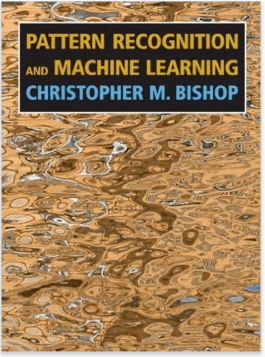This book is written by Christopher M Bishop. This book serves as a excellent reference for students keen to understand the use of statistical techniques in machine learning and pattern recognition. This books assumes the knowledge of linear algebra and multivariate calculus. It provides a comprehensive introduction to statistical pattern recognition techniques using practice exercises.

Because of the rapid development and active research in the field, there aren’t many printed and accessible books available on Deep Learning. However, I found that Yoshua Bengio, along with Ian Goodfellow and Aaron Courville is working on a book. You can check its recent developments here.

Neural Networks and Deep Learning: This book is written by Michael Neilson. It is available FREE online. If you are good at learning things at your own pace, I’d suggest you to read this book. There are just 6 Chapters. Every chapters goes in great detail of concepts related to deep learning using really nice illustrations.

## Blogs

Here are some of the best bet I have come across:

Beginners

Introduction to Neural Networks : This is Chapter 10 of Book, ‘The Nature of Code’. You’ll find the reading style simple and easy to comprehend. The author has explained neural network from scratch. Along with theory, you’ll also find codes(in python) to practice and apply them at your them. This not only would give you confidence to learn these concept, but would also allow you to experience their impact.

Hacker’s Guide to Neural Networks : Though, the codes in this blog are written in Javascript which you might not know. I’d still suggest you to refer it for the simplicity of theoretical concepts. This tutorial has very little math, but you’ll need lots of logic to comprehend and understand the following parts.

Intermediates

Recurrent Neural Network Part 1, Part 2, Part 3, Part 4 : After you are comfortable with basics of neural nets, it’s time to move to the next level. This is probably the best guide you would need to master RNN. RNN is a form of artificial neural network whose neurons send feedback signals to each other. I’d suggest you to follow the 4 parts religiously. It begins RNN from basics, followed by back propagation and its implementation.

Unreasonable Effectiveness of RNN: Consider this as an additional resource on RNN. If you are fond of seeking options, you might like to check this blog. It start with basic definition of RNN and goes all the way deep into building character models. This should help you give more hands on experience on implementing neural networks in various situations.

Backward Propogation Neural Network: Here you’ll find a simple explanation on the method of implementing backward propagation neural network. I’d suggest beginners to follow this blog and learn more about this concept. It will provide you a step by step approach for understanding neural networks deeply.

Deep Learning Tutorial by Stanford: This is by far the best tutorial/blog available on deep learning on internet. Having been recommended by many, it explains the complete science and mathematics behind every algorithm using easy to understand illustrations. This tutorial assumes basic knowledge of machine learning. Therefore, I’d suggest you to start with this tutorial after finishing Machine Learning course by Andrew Ng.

## Videos

Complete Tutorial on Neural Networks : This complete playlist of neural network tutorials should suffice your learning appetite. There were numerous videos I found, but offered a comprehensive learning like this one.

Note: In order to quickly get started, I’d recommend you to participate in Facial keypoint Detection Kaggle competition. Though, this competition ended long time back, you can still participate and practice. Moreover, you’ll also find benchmark solution for this competition. Here is the solution: Practice – Neural Nets. Get Going!

Deep Learning Lectures: Here is a complete series of lectures on Deep Learning from University of Oxford 2015. The instructor is Nando de Freitas. This tutorials covers a wide range of topics from linear models, logistic regression, regularization to recurrent neural nets. Instead of rushing through these videos, I’d suggest you to devote good amount of time and develop concrete understanding of these concepts. Start from Lecture 1.

Introduction to Deep Learning with Python: After learning the theoretical aspects of these algorithm, it’s now time to practice them using Python. This ~1 hour video is highly recommended to practice deep learning in python using theano.

Deep Learning Summer School, Montreal 2015: Here are the videos from Deep Learning Summer School, Montreal 2015. These videos covers advanced topics in Deep Learning. Hence, I wouldn’t recommend them to beginners. However, people with knowledge of machine learning must watch them. These videos will take your deep learning intellect to a new level. Needless to say, they are FREE to access.

## Research Papers

I could list here numerous paper published on Deep Learning, but that would have defeated the purpose. Hence, to highlight the best resources, I’ve listed some of the seminal papers in this field:

Deep Learning in Neural Networks

Introduction to Deep Learning

Deep Boltzmann Machines

Learning Deep Architectures for AI

Deep Learning of Representations: Looking Forward

Gradient based training for Deep Architechture

## End Notes

By now, I’m sure you have a lot of work carved out for yourself. I found them intimidating initially, but these videos and blogs totally helped me to regain my confidence. As said above, these are free resources and can be accessible from anywhere. If you are a beginner, I’d recommend you to start with Machine Learning course by Andrew Ng and read through blogs too.

I’ve tried to provide the best possible resources available on these topics at present. As mentioned before, I am not an expert on neural networks and machine learning (yet)! So it is quite possible that I missed out on some useful resource. Did I miss out any useful resource? May be! Please share your views / suggestions in the comments section below.

By

## Introduction

In my last article, I discussed the fundamentals of deep learning, where I explained the basic working of a artificial neural network. If you’ve been following this series, today we’ll become familiar with practical process of implementing neural network in Python (using Theano package).

I found various other packages also such as Caffe, Torch, TensorFlow etc to do this job. But, Theano is no less than and satisfactorily execute all the tasks. Also, it has multiple benefits which further enhances the coding experience in Python.

In this article, I’ll provide a comprehensive practical guide to implement Neural Networks using Theano. If you are here for just python codes, feel free to skip the sections and learn at your pace. And, if you are new to Theano, I suggest you to follow the article sequentially to gain complete knowledge.

Note:

2. If you don’t know python, start here.
3. If you don’t know deep learning, start here.1. Theano Overview
2. Implementing Simple expressions
3. Theano Variable Types
4. Theano Functions
5. Modeling a Single Neuron
6. Modeling a Two-Layer Networks

## 1. Theano Overview

In short, we can define Theano as:

• A programming language which runs on top of Python but has its own data structure which are tightly integrated with numpy
• A linear algebra compiler with defined C-codes at the backend
• A python package allowing faster implementation of mathematical expressions

As popularly known, Theano was developed at the University of Montreal in 2008. It is used for defining and evaluating mathematical expressions in general.

Theano has several features which optimize the processing time of expressions. For instance it modifies the symbolic expressions we define before converting them to C codes. Examples:

• It makes the expressions faster, for instance it will change { (x+y) + (x+y) } to { 2*(x+y) }
• It makes expressions more stable, for instance it will change { exp(a) / exp(a).sum(axis=1) } to { softmax(a) }

Below are some powerful advantages of using Theano:

1. It defines C-codes for different mathematical expressions.
2. The implementations are much faster as compared to some of the python’s default implementations.
3. Due to fast implementations, it works well in case of high dimensionality problems.
4. It allows GPU implementation which works blazingly fast specially for problems like deep learning.

Let’s now focus on Theano (with example) and try to understand it as a programming language.

## 2. Implementing Simple Expressions

Lets start by implementing a simple mathematical expression, say a multiplication in Theano and see how the system works. In later sections, we will take a deep dive into individual components. The general structure of a Theano code works in 3 steps:

1. Define variables/objects
2. Define a mathematical expression in the form of a function
3. Evaluate expressions by passing values

Lets look at the following code for simply multiplying 2 numbers:

#### Step 0: Import libraries

import numpy as np
import theano.tensor as T
from theano import function

Here, we have simply imported 2 key functions of theano – tensor and function.

#### Step 1: Define variables

a = T.dscalar('a')
b = T.dscalar('b')

Here 2 variables are defined. Note that we have used Theano tensor object type here. Also, the arguments passed to dscalar function are just name of tensors which are useful while debugging. They code will work even without them.

#### Step 2: Define expression

c = a*b
f = function([a,b],c)

Here we have defined a function f which has 2 arguments:

1. Inputs [a,b]: these are inputs to system
2. Output c: this has been previously defined

#### Step 3: Evaluate Expression

f(1.5,3)Now we are simply calling the function with the 2 inputs and we get the output as a multiple of the two. In short, we saw how we can define mathematical expressions in Theano and evaluate them. Before we go into complex functions, lets understand some inherent properties of Theano which will be useful in building neural networks.

## 3. Theano Variable Types

Variables are key building blocks of any programming language. In Theano, the objects are defined as tensors. A tensor can be understood as a generalized form of a vector with dimension t. Different dimensions are analogous to different types:

• t = 0: scalar
• t = 1: vector
• t = 2: matrix
• and so on..

Watch this interesting video to get a deeper level of intuition into vectors and tensors.

These variables can be defined similar to our definition of ‘dscalar’ in the above code. The various keywords for defining variables are:

• byte: bscalar, bvector, bmatrix, brow, bcol, btensor3, btensor4
• 16-bit integers: wscalar, wvector, wmatrix, wrow, wcol, wtensor3, wtensor4
• 32-bit integers: iscalar, ivector, imatrix, irow, icol, itensor3, itensor4
• 64-bit integers: lscalar, lvector, lmatrix, lrow, lcol, ltensor3, ltensor4
• float: fscalar, fvector, fmatrix, frow, fcol, ftensor3, ftensor4
• double: dscalar, dvector, dmatrix, drow, dcol, dtensor3, dtensor4
• complex: cscalar, cvector, cmatrix, crow, ccol, ctensor3, ctensor4

Now you understand that we can define variables with different memory allocations and dimensions. But this is not an exhaustive list. We can define dimensions higher than 4 using a generic TensorType class. You’ll find more details here.

Please note that variables of these types are just symbols. They don’t have a fixed value and are passed into functions as symbols. They only take values when a function is called. But, we often need variables which are constants and which we need not pass in all the functions. For this Theano provides shared variables. These have a fixed value and are not of the types discussed above. They can be defined as numpy data types or simple constants.

Lets take an example. Suppose, we initialize a shared variable as 0 and use a function which:

• takes an input
• adds the input to the shared variable
• returns the square of shared variable

This can be done as:

from theano import shared
x = T.iscalar('x')
sh = shared(0)
f = function([x], sh**2, updates=[(sh,sh+x)])

Note that here function has an additional argument called updates. It has to be a list of lists or tuples, each containing 2 elements of form (shared_variable, updated_value). The output for 3 subsequent runs is:You can see that for each run, it returns the square of the present value, i.e. the value before updating. After the run, the value of shared variable gets updated. Also, note that shared variables have 2 functions “get_value()” and “set_value()” which are used to read and modify the value of shared variables.

## 4. Theano Functions

Till now we saw the basic structure of a function and how it handles shared variables. Lets move forward and discuss couple more things we can do with functions:

#### Return Multiple Values

We can return multiple values from a function. This can be easily done as shown in following example:

a = T.dscalar('a')
f = function([a],[a**2, a**3])
f(3)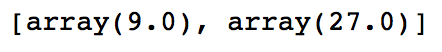We can see that the output is an array with the square and cube of the number passed into the function.

Gradient computation is one of the most important part of training a deep learning model. This can be done easily in Theano. Let’s define a function as the cube of a variable and determine its gradient.

x = T.dscalar('x')
y = x**3
f = function([x],qy)
f(4)

This returns 48 which is 3x2 for x=4. Lets see how Theano has implemented this derivative using the pretty-print feature as following:

from theano import pp  #pretty-print
print(pp(qy))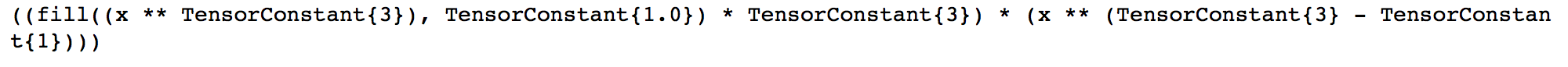In short, it can be explained as: fill(x3,1)*3*x3-1 You can see that this is exactly the derivative of x3. Note that fill(x3,1) simply means to make a matrix of same shape as x3 and fill it with 1. This is used to handle high dimensionality input and can be ignored in this case.

We can use Theano to compute Jacobian and Hessian matrices as well which you can find here.

There are various other aspects of Theano like conditional and looping constructs. You can go into further detail using following resources:

## 5. Modeling a Single Neuron

Lets start by modeling a single neuron.

Note that I will take examples from my previous article on neuron networks here. If you wish to go in the detail of how these work, please read this article. For modeling a neuron, lets adopt a 2 stage process:

1. Implement Feed Forward Pass
• take inputs and determine output
• use the fixed weights for this case
2. Implement Backward Propagation

Lets implement an AND gate for this purpose.

### Feed Forward Pass

An AND gate can be implemented as: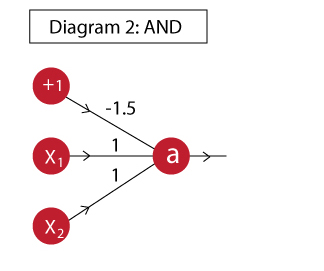Now we will define a feed forward network which takes inputs and uses the shown weights to determine the output. First we will define a neuron which computes the output a.

import theano
import theano.tensor as T
from theano.ifelse import ifelse
import numpy as np

#Define variables:
x = T.vector('x')
w = T.vector('w')
b = T.scalar('b')

#Define mathematical expression:
z = T.dot(x,w)+b
a = ifelse(T.lt(z,0),0,1)

neuron = theano.function([x,w,b],a)

I have simply used the steps we saw above. If you are not sure how this expression works, please refer to the neural networks article I have referred above. Now let’s test out all values in the truth table and see if the AND function has been implemented as desired.

#Define inputs and weights
inputs = [
[0, 0],
[0, 1],
[1, 0],
[1, 1]
]
weights = [ 1, 1]
bias = -1.5

#Iterate through all inputs and find outputs:
for i in range(len(inputs)):
t = inputs[i]
out = neuron(t,weights,bias)
print 'The output for x1=%d | x2=%d is %d' % (t,t,out)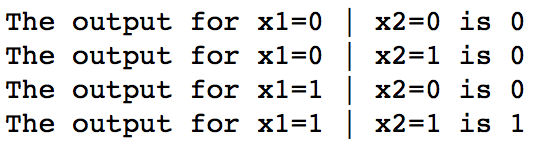Note that, in this case we had to provide weights while calling the function. However, we will be required to update them while training. So, its better that we define them as a shared variable. The following code implements w as a shared variable. Try this out and you’ll get the same output.

import theano
import theano.tensor as T
from theano.ifelse import ifelse
import numpy as np

#Define variables:
x = T.vector('x')
w = theano.shared(np.array([1,1]))
b = theano.shared(-1.5)

#Define mathematical expression:
z = T.dot(x,w)+b
a = ifelse(T.lt(z,0),0,1)

neuron = theano.function([x],a)

#Define inputs and weights
inputs = [
[0, 0],
[0, 1],
[1, 0],
[1, 1]
]

#Iterate through all inputs and find outputs:
for i in range(len(inputs)):
t = inputs[i]
out = neuron(t)
print 'The output for x1=%d | x2=%d is %d' % (t,t,out)

Now the feedforward step is complete.

### Backward Propagation

Now we have to modify the above code and perform following additional steps:

1. Determine the cost or error based on true output
3. Update the weights using this gradient

Lets initialize the network as follow:

#Gradient
import theano
import theano.tensor as T
from theano.ifelse import ifelse
import numpy as np
from random import random

#Define variables:
x = T.matrix('x')
w = theano.shared(np.array([random(),random()]))
b = theano.shared(1.)
learning_rate = 0.01

#Define mathematical expression:
z = T.dot(x,w)+b
a = 1/(1+T.exp(-z))

Note that, you will notice a change here as compared to above program. I have defined x as a matrix here and not a vector. This is more of a vectorized approach where we will determine all the outputs together and find the total cost which is required for determining the gradients.

You should also keep in mind that I am using the full-batch gradient descent here, i.e. we will use all training observations to update the weights.

Let’s determine the cost as follows:

a_hat = T.vector('a_hat') #Actual output
cost = -(a_hat*T.log(a) + (1-a_hat)*T.log(1-a)).sum()

In this code, we have defined a_hat as the actual observations. Then we determine the cost using a simple logistic cost function since this is a classification problem. Now lets compute the gradients and define a means to update the weights.

dw,db = T.grad(cost,[w,b])

train = function(
inputs = [x,a_hat],
outputs = [a,cost],
[w, w-learning_rate*dw],
[b, b-learning_rate*db]
]
)

In here, we are first computing gradient of the cost w.r.t. the weights for inputs and bias unit. Then, the train function here does the weight update job. This is an elegant but tricky approach where the weights have been defined as shared variables and the updates argument of the function is used to update them every time a set of values are passed through the model.

#Define inputs and weights
inputs = [
[0, 0],
[0, 1],
[1, 0],
[1, 1]
]
outputs = [0,0,0,1]

#Iterate through all inputs and find outputs:
cost = []
for iteration in range(30000):
pred, cost_iter = train(inputs, outputs)
cost.append(cost_iter)

#Print the outputs:
print 'The outputs of the NN are:'
for i in range(len(inputs)):
print 'The output for x1=%d | x2=%d is %.2f' % (inputs[i],inputs[i],pred[i])

#Plot the flow of cost:
print '\nThe flow of cost during model run is as following:'
import matplotlib.pyplot as plt
%matplotlib inline
plt.plot(cost)Here we have simply defined the inputs, outputs and trained the model. While training, we have also recorded the cost and its plot shows that our cost reduced towards zero and then finally saturated at a low value. The output of the network also matched the desired output closely. Hence, we have successfully implemented and trained a single neuron.

## 6. Modeling a Two-Layer Neural Network

I hope you have understood the last section. If not, please do read it multiple times and proceed to this section. Along with learning Theano, this will enhance your understanding of neural networks on the whole.

Lets consolidate our understanding by taking a 2-layer example. To keep things simple, I’ll take the XNOR example like in my previous article. If you wish to explore the nitty-gritty of how it works, I recommend reading the previous article.

The XNOR function can be implemented as: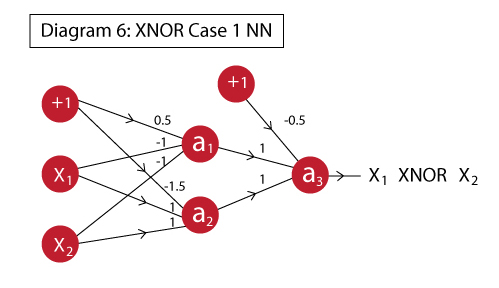As a reminder, the truth table of XNOR function is: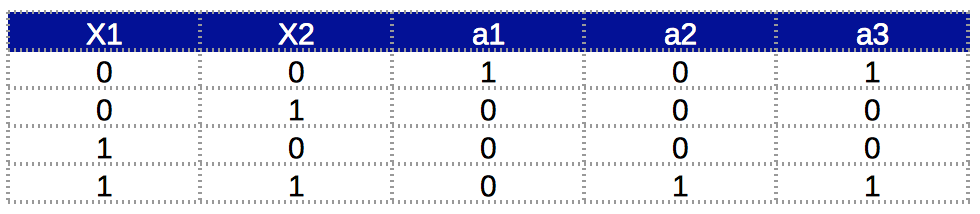Now we will directly implement both feed forward and backward at one go.

### Step 1: Define variables

import theano
import theano.tensor as T
from theano.ifelse import ifelse
import numpy as np
from random import random

#Define variables:
x = T.matrix('x')
w1 = theano.shared(np.array([random(),random()]))
w2 = theano.shared(np.array([random(),random()]))
w3 = theano.shared(np.array([random(),random()]))
b1 = theano.shared(1.)
b2 = theano.shared(1.)
learning_rate = 0.01

In this step we have defined all the required variables as in the previous case. Note that now we have 3 weight vectors corresponding to each neuron and 2 bias units corresponding to 2 layers.

### Step 2: Define mathematical expression

a1 = 1/(1+T.exp(-T.dot(x,w1)-b1))
a2 = 1/(1+T.exp(-T.dot(x,w2)-b1))
x2 = T.stack([a1,a2],axis=1)
a3 = 1/(1+T.exp(-T.dot(x2,w3)-b2))

Here we have simply defined mathematical expressions for each neuron in sequence. Note that here an additional step was required where x2 is determined. This is required because we want the outputs of a1 and a2 to be combined into a matrix whose dot product can be taken with the weights vector.

Lets explore this a bit further. Both a1 and a2 would return a vector with 4 units. So if we simply take an array [a1, a2] then we’ll obtain something like [ [a11,a12,a13,a14], [a21,a22,a23,a24] ]. However, we want this to be [ [a11,a21], [a12,a22], [a13,a23], [a14,a24] ]. The stacking function of Theano does this job for us.

### Step 3: Define gradient and update rule

a_hat = T.vector('a_hat') #Actual output
cost = -(a_hat*T.log(a3) + (1-a_hat)*T.log(1-a3)).sum()

train = function(
inputs = [x,a_hat],
outputs = [a3,cost],
[w1, w1-learning_rate*dw1],
[w2, w2-learning_rate*dw2],
[w3, w3-learning_rate*dw3],
[b1, b1-learning_rate*db1],
[b2, b2-learning_rate*db2]
]
)

This is very similar to the previous case. The key difference being that now we have to determine the gradients of 3 weight vectors and 2 bias units and update them accordingly.

### Step 4: Train the model

inputs = [
[0, 0],
[0, 1],
[1, 0],
[1, 1]
]
outputs = [1,0,0,1]

#Iterate through all inputs and find outputs:
cost = []
for iteration in range(30000):
pred, cost_iter = train(inputs, outputs)
cost.append(cost_iter)

#Print the outputs:
print 'The outputs of the NN are:'
for i in range(len(inputs)):
print 'The output for x1=%d | x2=%d is %.2f' % (inputs[i],inputs[i],pred[i])

#Plot the flow of cost:
print '\nThe flow of cost during model run is as following:'
import matplotlib.pyplot as plt
%matplotlib inline
plt.plot(cost)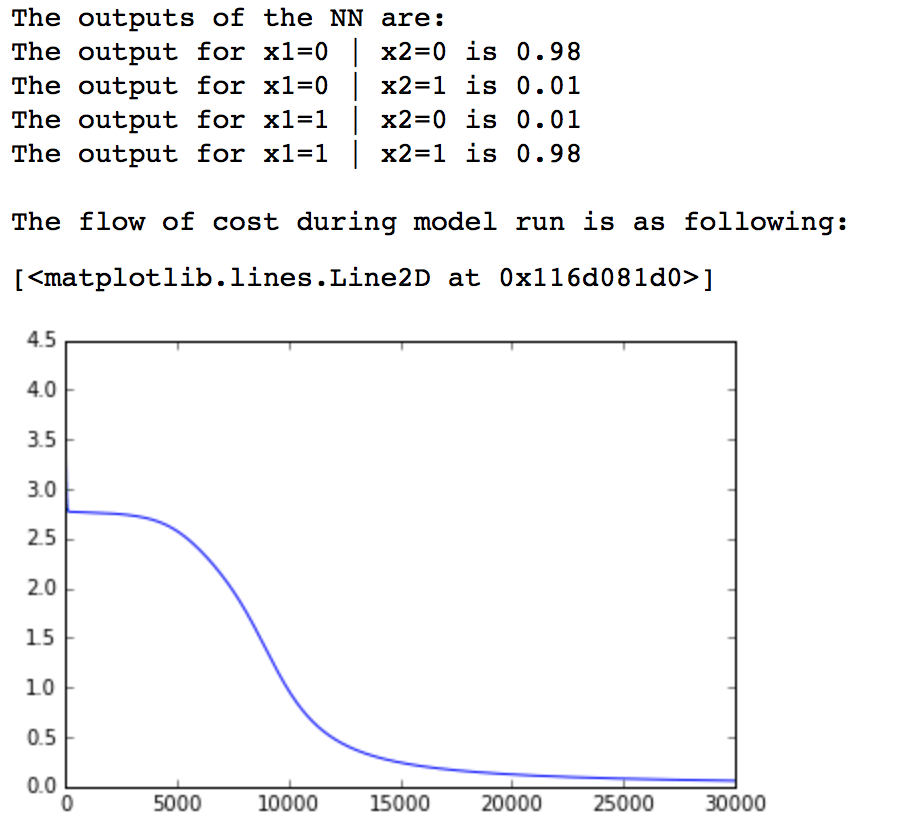We can see that our network has successfully learned the XNOR function. Also, the cost of the model has reduced to reasonable limit. With this, we have successfully implemented a 2-layer network.

## End Notes

In this article, we understood the basics of Theano package in Python and how it acts as a programming language. We also implemented some basic neural networks using Theano. I am sure that implementing Neural Networks on Theano will enhance your understanding of NN on the whole.

If hope you have been able to follow till this point, you really deserve a pat on your back. I can understand that Theano is not a traditional plug and play system like most of sklearn’s ML models. But the beauty of neural networks lies in their flexibility and an approach like this will allow you a high degree of customization in models. Some high-level wrappers of Theano do exist like Keras and Lasagne which you can check out. But I believe knowing the core of Theano will help you in using them.